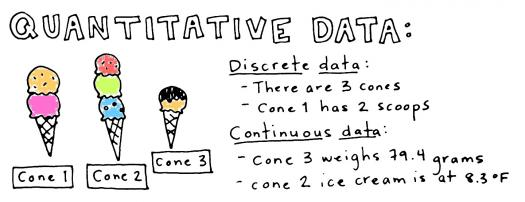# Summarizing Quantitative Data Assessment Test II

10 Questions | Total Attempts: 141SettingsQuantitative data is a type of data that can be measured and written down, measuring information about quantities. Take this assessment test to assess your knowledge of summarizing quantitative data.

• 1.
Information that can be measured and written down with numbers is termed...
• A.

Qualitative Data

• B.

Quantitative data

• C.

Figures

• D.

• 2.
Data that are measures of types and may be represented by a name or symbol are said to be...
• A.

Quantitative

• B.

Qualitative

• C.

Number

• D.

Figure

• 3.
A count that cannot be made precise is referred to as...
• A.

Count data

• B.

Real figure

• C.

Discrete data

• D.

Real data

• 4.
Which is a type of quantitative data?
• A.

Continuous data

• B.

Real data

• C.

Number data

• D.

Outliner

• 5.
Which is an example of quantitative data?
• A.

Light

• B.

Type

• C.

Color

• D.

Height

• 6.
Which one of these is a method for collecting qualitative data?
• A.

Observation

• B.

Writing

• C.

Picture

• D.

Drawing

• 7.
Qualitative data does one of these.
• A.

Writes

• B.

Describes

• C.

Draws

• D.

Makes

• 8.
Which is a type of data?
• A.

Picture

• B.

Tree

• C.

Figure

• D.

Ordinal

• 9.
The systematic empirical investigation of observable phenomena via statistical or mathematical techniques is called...
• A.

Research

• B.

Quantitative research

• C.

Qualitative research

• D.

Final research

• 10.
Which of the following is a type of data?
• A.

Nominal

• B.

Round

• C.

Outlier

• D.

Wrong

Related TopicsBack to top